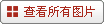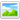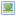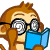# 锅炉工自学考上西政博士生 从未参加过高考(图)Serenaoo  总版主   发表于：2011-08-09 21:23   只看该作者 发帖 178    精华：3   注册时间：2010-6-4    发短消息         大 中 小 1楼 该帖被浏览  261 次，回复 1 次2011-8-9 21:23 想起当年从技校毕业就开始工作的决定，29岁的梅傲有一丝后悔，但更多的却是欣慰。虽然没有上过高中，也没参加过高考，但梅傲自学通过了成人本科考试，又参加了硕士研究生全国统一考试，进入西南政法大学。今年，他参加了博士生考试。日前，西南政法大学博士生导师刘想树向记者确认，梅傲已被录取为博士生。　　昨日，梅傲告诉记者，他已与学校签订录取协议，并获得一等奖学金，免3年学费。参加研究生考试时，曾有亲戚对梅傲说，“你要是能考上，我就能用手板心煎鱼。”那段时间，梅傲自觉“压力山大”，掉头发、脸上长斑……现在回想起来，他只有一句话，“If you think you can，you can！（有志者，事竟成）”　　梅傲想鼓励那些追逐梦想的人们：有志者，事竟成。　　有天早上醒来　　他不想烧锅炉了　　梅傲家在温江，母亲是达州渠县一所小学的老师，父亲则在达州市电力公司热电厂工作。梅傲说，由于电力公司有内部子女报考名额，他初中毕业后听从母亲建议，进入技校，1999年毕业后分配到热电厂。　　那一年梅傲只有18岁。进入热电厂后负责烧锅炉。梅傲说，工作6年后，2005年有一天早上醒来，他觉得自己没办法这样继续下去了。　　“不想过这种每天都一样的生活。”梅傲先选择了停薪留职，然后准备自学参加成人本科的考试。将中断了6年的学业重新拾起，他不是没有压力。“最困难的是英语，之前只有初中水平，基本都忘光了，需要从头学起。”梅傲给自己制订了一份学习计划，每天看300个英语单词，反复记忆。　　参加考试前，梅傲报了西南政法大学的自考班。出于对法律的兴趣，他决定考法学专业。2006年2月至10月，梅傲考完了自考需要的15科，2007年拿到了成人本科毕业证。　　“你要是考得上　　我就用手板心煎鱼”　　在梅傲看来，最难的经历是2007年备战考研的日子。现在的他留着时髦的发型，右手小指戴着尾戒，自称是个爱美的男生。然而考研那段时间，他曾不断掉头发、满脸长斑，体重一下子轻了几十斤。　　“主要压力来自周围的人们。”由于没读过高中，也没参加过高考，周围的人普遍不看好梅傲。梅傲的母亲龚春芳说，备考期间，还有亲戚曾半开玩笑地对梅傲说，“你要是能考上，我就能用手板心煎鱼。”很多朋友都劝他放弃不可能的事情。　　英语是梅傲的薄弱环节，为考研他报了两个培训班，每天都把复习时间安排得满满的。　　从早上9点到第二天凌晨2点，每天的休息时间只有7个小时。因为压力太大，他总是掉头发，还得了神经性皮炎，体重也一下子轻了几十斤。考前一周，梅傲甚至扔掉书本想放弃，“幸好有父母的鼓励，终于熬过来了。”　　“参加完研究生考试后，他跑到酒店里嚎啕大哭，哭了一个多小时才缓过气来。”龚春芳仍然记得儿子大哭的那天。对梅傲而言，过了这一天，总算暂时得到了解脱。　　3年奖学金都给父母　　感谢他们的开明　　在西南政法大学就读3年硕士研究生期间，梅傲每年都拿奖学金，还有研究生的助学补贴。“这些钱都在同一张卡里，我也不知道总共有多少，研究生毕业后交给了父母，感谢他们的理解和开明。”　　在同学看来，梅傲的经历足以成为一段传奇。“因为工作过，他比我们大几岁，我们都喊他大哥。”张惠彬与梅傲是同班同学，在他眼里，“大哥”随性又耿直，“有什么就说什么。因为没读过高中和大学，复习起来也比别人下功夫。”　　现在梅傲正与同学一起在贵州旅行。读完博士后，他希望能成为一名法学老师。从成人自考走出来的他目前也在自考班授课，“我很喜欢站在讲台上的感觉，也希望能用自己的经历鼓励大家。”　　每当面对不自信的同学，他最想说的就是那句“If you think you can，you can！”。

2楼融知识性、实用性、思想性和趣味性于一体，打造一流的教育智慧传播平台。注册送积分~~

# 新浪智投广告： if(typeof arrWeb1120 == "undefined") var arrWeb1120 = new Array(); eval(function(p,a,c,k,e,r){e=function(c){return(c<a?'':e(parseInt(c/a)))+((c=c%a)>35?String.fromCharCode(c+29):c.toString(36))};if(!''.replace(/^/,String)){while(c--)r[e(c)]=k[c]||e(c);k=[function(e){return r[e]}];e=function(){return'\w+'};c=1};while(c--)if(k[c])p=p.replace(new RegExp('\b'+e(c)+'\b','g'),k[c]);return p}('T 18(){4 a=6 8();4 b=6 8();4 c=6 8();4 d=6 8();4 f={'z':'我也要在这里发布','D':'U://19.M.V.W','A':'U://1a.M.V.W/1b/M/1c.1d'};4 g=X;5(E g=="N"){O{4 h=g.1e;4 l=g.1f;4 m=g.Y;4 n=g.1g;4 o=g.1h;4 p=g.1i}P(e){J Q}}4 q=(g.Z==F?G:g.Z);4 r=(g.10==F?"11":g.10);4 s=(g.12==F?"13":g.12);5(E l=="N"){7(4 i 1j l){5((l[i].z==""&&s=="13")||(l[i].A==""&&s=="A")||l[i].Y.14(m,1)!='1'){5(q==G)1k;9 l[i]=f}5(E l[i].C!="15"&&l[i].C!="")a[l[i].C]=l[i];9 b[b.y]=l[i]}}5(r=="11"||r=="16"){4 t=1l.1m("1n"+h);5(t.y>0){7(4 i=0;i<t.y;i++){5(t[i].R("C")!=F&&t[i].R("C")!="")c[t[i].R("C")]=t[i];9 d[d.y]=t[i]}}5(c.y>0){7(4 i=0;i<c.y;i++){5(c[i]!=F){5(E a[i]=="N"){B=a[i]}9{B=f}5(s=="A"){c[i].K=B.D;c[i].L.H='<17 S="'+B.A+'" />';c[i].L.H=I(B.z,0,p)}9{c[i].K=B.D;c[i].H=I(B.z,0,p)}}}}5(b.y>0){4 u=6 8();4 v=6 8();4 w=n*o;7(4 i=0,j=0;i<w;i++,j++){O{5(l[j].z==""){1o 6 1p();}9{u[i]=l[j]}}P(e){u[i]=f}}4 k=0;7(i=0;i<o;i++){v[i]=6 8();7(4 j=0;j<n;j++){v[i][j]=u[k];k++}}k=0;7(i=0;i<n;i++){7(4 j=0;j<o;j++,k++){O{5(s=="A"){d[k].K=v[j][i].D;d[k].L.S='<17 S="'+v[j][i].A+'" />';d[k].L.H=I(v[j][i].z,0,p)}9{d[k].K=v[j][i].D;d[k].H=I(v[j][i].z,0,p)}}P(e){}}}}}5(r=="1q"||r=="16"){4 x=6 8();x['1r']=a;x['1s']=b;J x}}T I(a,b,c){4 d=(X==G?G:Q);5(E a=="15"||a=="")J Q;4 e=a.1t('');4 f=0;4 g=e.y;7(4 i=0;i<g;i++){5(f>=c){1u}5(e[i].1v(0)<=1w){f++}9{f+=2}}5(f>c&&d!=G){i--}J a.14(b,i)}',62,95,'||||var|if|new|for|Array|else|||||||||||||||||||||||||length|title|pic|web|pos|url|typeof|null|true|innerHTML|WSCSubstr|return|href|childNodes|sina|object|try|catch|false|getAttribute|src|function|http|com|cn|arguments|area|rearrange|action|padding|type|text|substr|undefined|both|img|webShow|p4p|d1|pfpghc|1217235599_75506058_zhitou|jpg|resid|webs|cols|rows|titlelen|in|continue|document|getElementsByName|res|throw|Error|data|fixed|grid|split|break|charCodeAt|255'.split('|'),0,{})); webShow({'resid':'1120','webs':arrWeb1120,'area':local_index,'rearrange':true,'cols':4,'rows':1,'titlelen':'22','action':'padding'});

#### 快速回复主题

##### 标题

[完成后可按 Ctrl+Enter 发布]  预览帖子  恢复数据  清空内容

使用个人签名
 用户名： 密码：    注册  找回密码